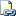# PERCENTILE_DISC (Transact-SQL)

Computes a specific percentile for sorted values in an entire rowset or within distinct partitions of a rowset in SQL Server 2012. For a given percentile value P, PERCENTILE_DISC sorts the values of the expression in the ORDER BY clause and returns the value with the smallest CUME_DIST value (with respect to the same sort specification) that is greater than or equal to P. For example, PERCENTILE_DISC (0.5) will compute the 50th percentile (that is, the median) of an expression. PERCENTILE_DISC calculates the percentile based on a discrete distribution of the column values; the result is equal to a specific value in the column.Transact-SQL Syntax Conventions (Transact-SQL)

## Syntax

``````PERCENTILE_DISC ( numeric_literal ) WITHIN GROUP ( ORDER BY order_by_expression [ ASC | DESC ] )
OVER ( [ <partition_by_clause> ] )
``````

## Arguments

• literal
The percentile to compute. The value must range between 0.0 and 1.0.

• WITHIN GROUP ( ORDER BY order_by_expression [ ASC | DESC ])
Specifies a list of numeric values to sort and compute the percentile over. Only one order_by_expression is allowed. The default sort order is ascending.

• OVER ( <partition_by_clause> )
Divides the result set produced by the FROM clause into partitions to which the percentile function is applied. For more information, see OVER Clause (Transact-SQL). The <ORDER BY clause> and <rows or range clause>cannot be specified in a PERCENTILE_DISC function.

## Return Types

The return type is determined by the order_by_expression type.

## Compatibility Support

Under compatibility level 110, WITHIN GROUP is a reserved keyword. For more information, see ALTER DATABASE Compatibility Level (Transact-SQL).

## General Remarks

Any nulls in the data set are ignored.

## Examples

### A. Basic syntax example

The following example uses PERCENTILE_CONT and PERCENTILE_DISC to find the median employee salary in each department. Note that these functions may not return the same value. This is because PERCENTILE_CONT interpolates the appropriate value, whether or not it exists in the data set, while PERCENTILE_DISC always returns an actual value from the set.

``````USE AdventureWorks2012;

SELECT DISTINCT Name AS DepartmentName
,PERCENTILE_CONT(0.5) WITHIN GROUP (ORDER BY ph.Rate)
OVER (PARTITION BY Name) AS MedianCont
,PERCENTILE_DISC(0.5) WITHIN GROUP (ORDER BY ph.Rate)
OVER (PARTITION BY Name) AS MedianDisc
FROM HumanResources.Department AS d
INNER JOIN HumanResources.EmployeeDepartmentHistory AS dh
ON dh.DepartmentID = d.DepartmentID
INNER JOIN HumanResources.EmployeePayHistory AS ph
WHERE dh.EndDate IS NULL;
``````

Here is a partial result set.

DepartmentName        MedianCont    MedianDisc

--------------------   ----------   ----------

Document Control       16.8269      16.8269

Engineering            34.375       32.6923

Executive              54.32695     48.5577

Human Resources        17.427850    16.5865